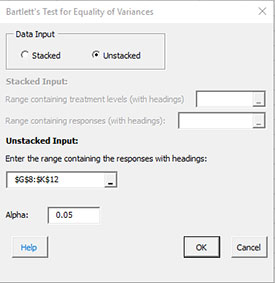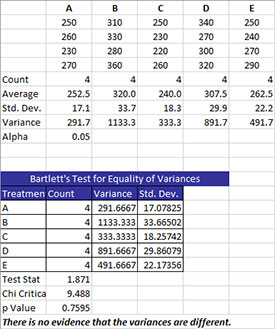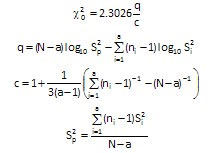# Bartlett’s Test for Equality of Variances HelpBartlett’s Test is used to determine if the variances from multiple treatments (or processes) are the same.

There are two options for entering the data: stacked or unstacked. Stacked data have each treatment in a single column. Unstacked data have the treatment labels in one column and the results in the adjacent column. The example below uses unstacked data.

Five different treatments (A to E) were used to control the weight of a coating in grams. There were four samples for each treatment. We want to use Bartlett’s test method to determine if there are any significant differences in the treatment variances.

1. Enter the data as shown below. The column headings represent the treatment labels with the responses for each treatment under the label. The number of results for each treatment do not have to be equal. The data can be downloaded at this link.2. Select the data and the column headings.

3. Select “Multiple Processes” from the “Statistical Tools” panel in the SPC for Excel ribbon.

4. Select the “Bartlett’s Test for Equality of Variances” option. Select OK and the input form below is displayed.• Data Input: there are two options: stacked and unstacked. If the range selected on the worksheet contains more than two columns, the default is unstacked; this can be changed. For more information on stacked versus unstacked data, click here.
• Stacked Option: these are the ranges to be entered:
• Range containing the treatment levels.
• Range containing the responses.
• Unstacked Option:
• The data must be columns with the first cell in each column representing the treatment level.
• Alpha: this is the confidence level; 1-alpha is the confidence interval; default is 0.05 for 95% confidence.
• Select OK to generate the results.
• Select Cancel to end the program.

Bartlett’s Test for Equality of Variances Output

The output for Bartlett’s test for equality of variances is shown below.A new worksheet is added to the workbook. The top part of the worksheet contains the data in stacked format along with some statistics for each treatment including count, average, standard deviation, and variance. The value of alpha is also given. The bottom part of the worksheet contains the information from Bartlett’s test.

The treatments are listed along with the variance and standard deviation. The following three statistics are given:

• Test Stat: this is the value of the chi-square test statistic (where N = total number of observations, a = number of treatments, and Si2 is the variance of the ith treatment):• Chi Critical: this the critical chi-square value based on alpha and the degrees of freedom (which is the number of treatments – 1); if the test stat > chi critical, reject the null hypothesis
• p value: Reject the null hypothesis if p value < alpha.